CBSE Class 10 Sample Paper for 2018 Boards

Class 10
Solutions of Sample Papers for Class 10 Boards

### If sin θ + cos θ = √2, then evaluate : tan θ + cot θ .

This is a question of CBSE Sample Paper - Class 10 - 2017/18.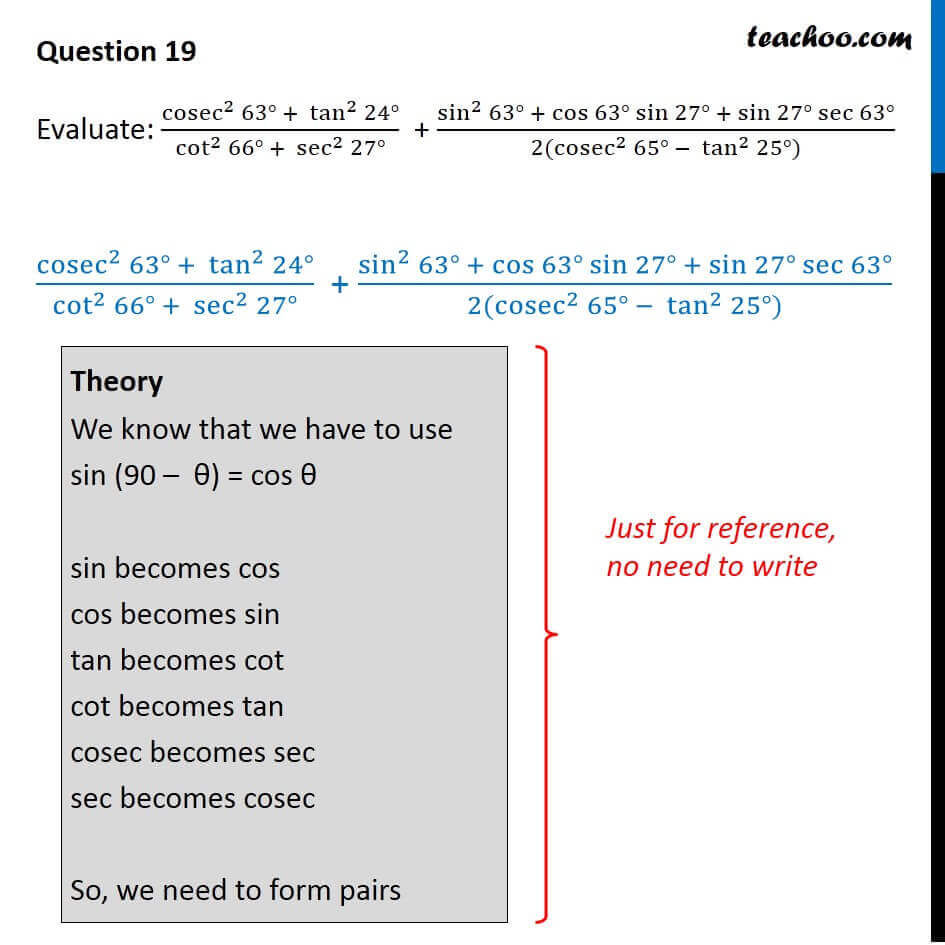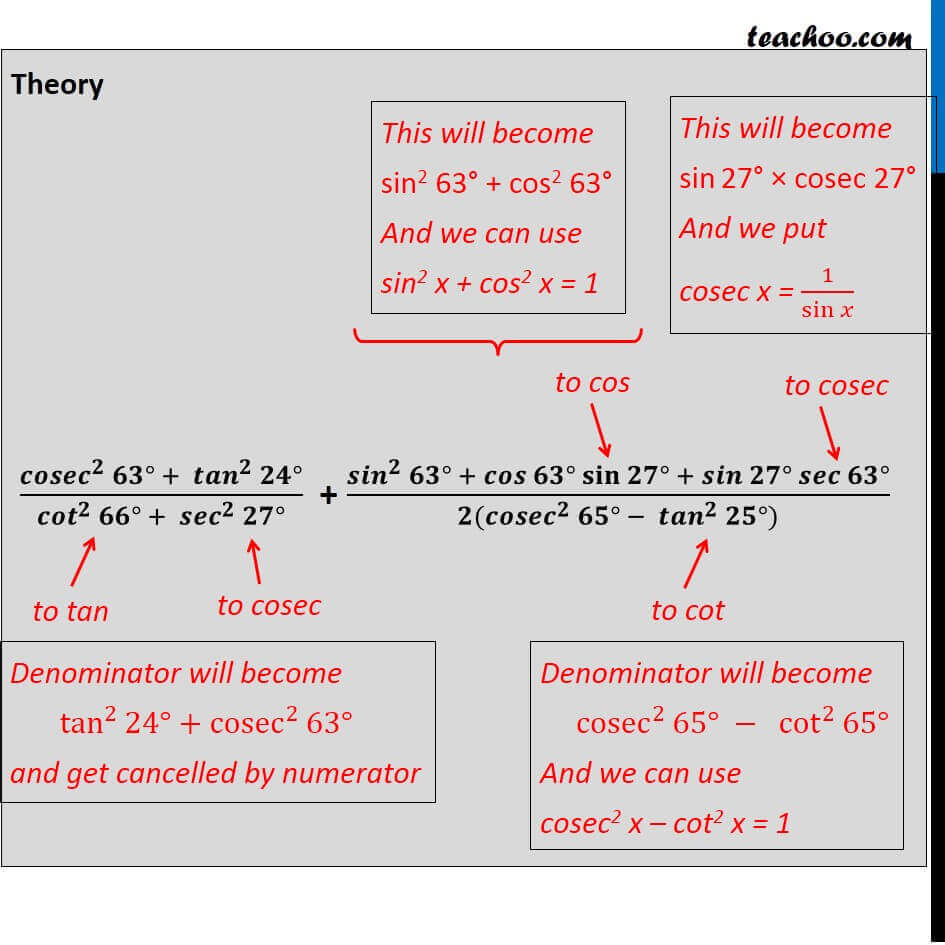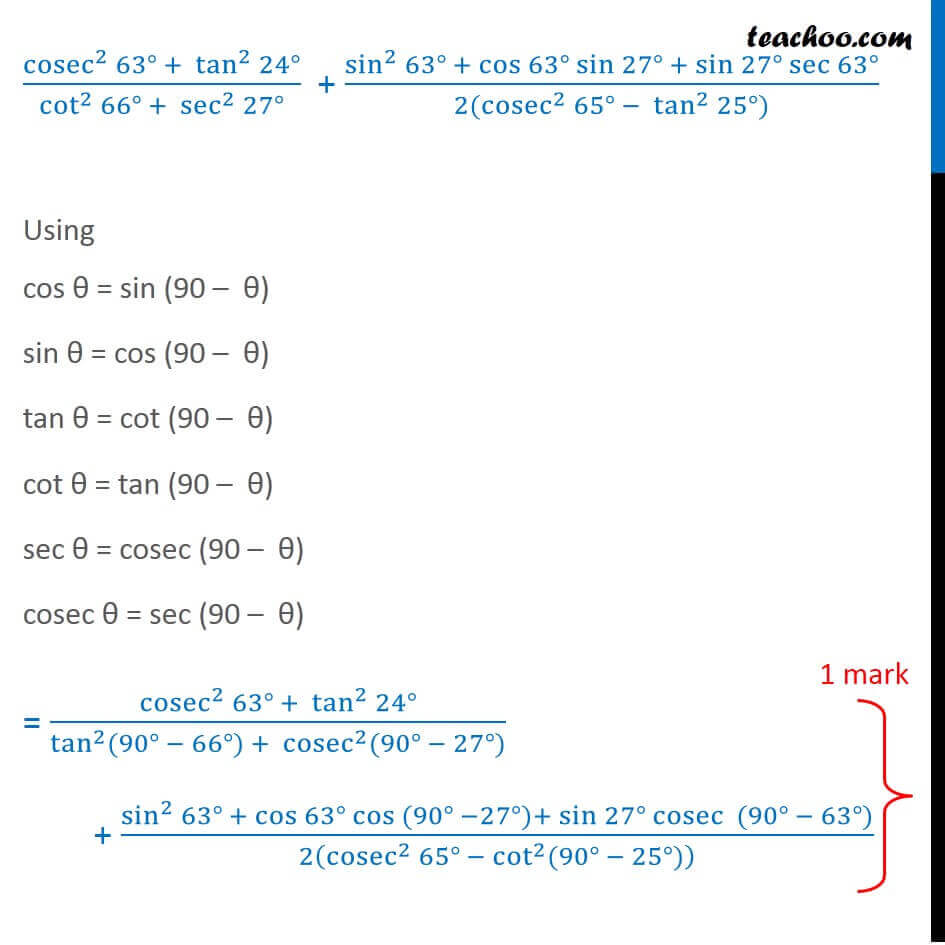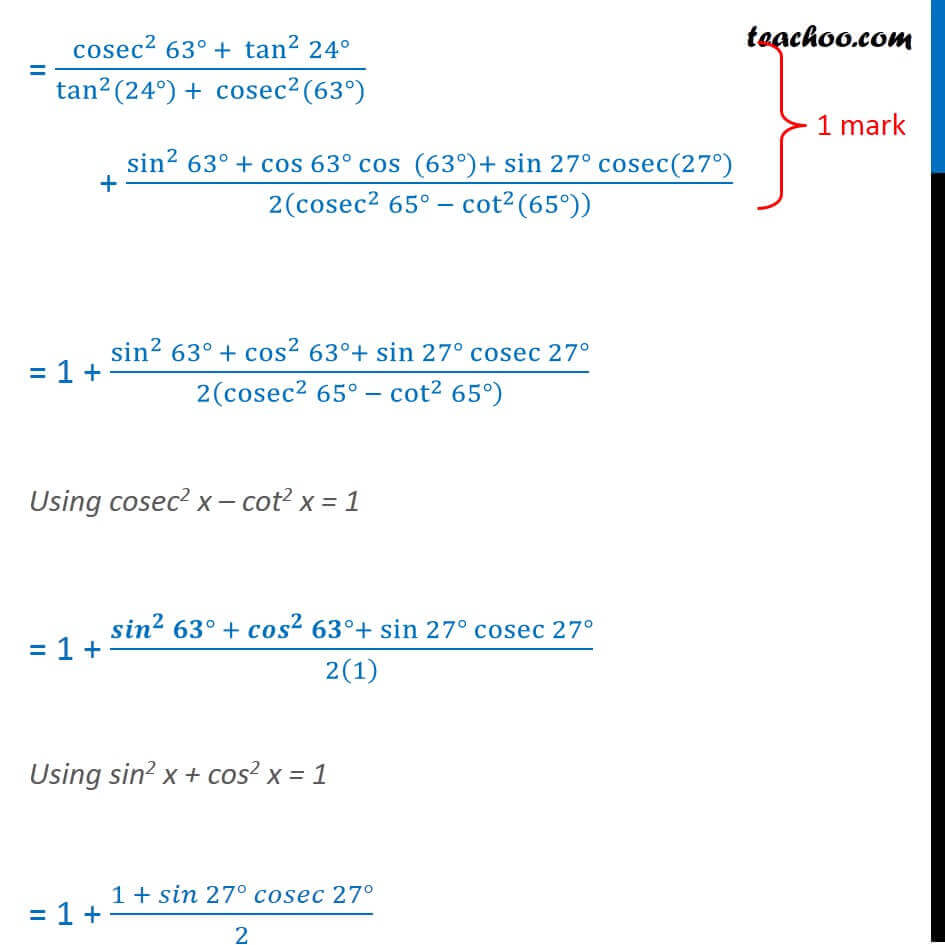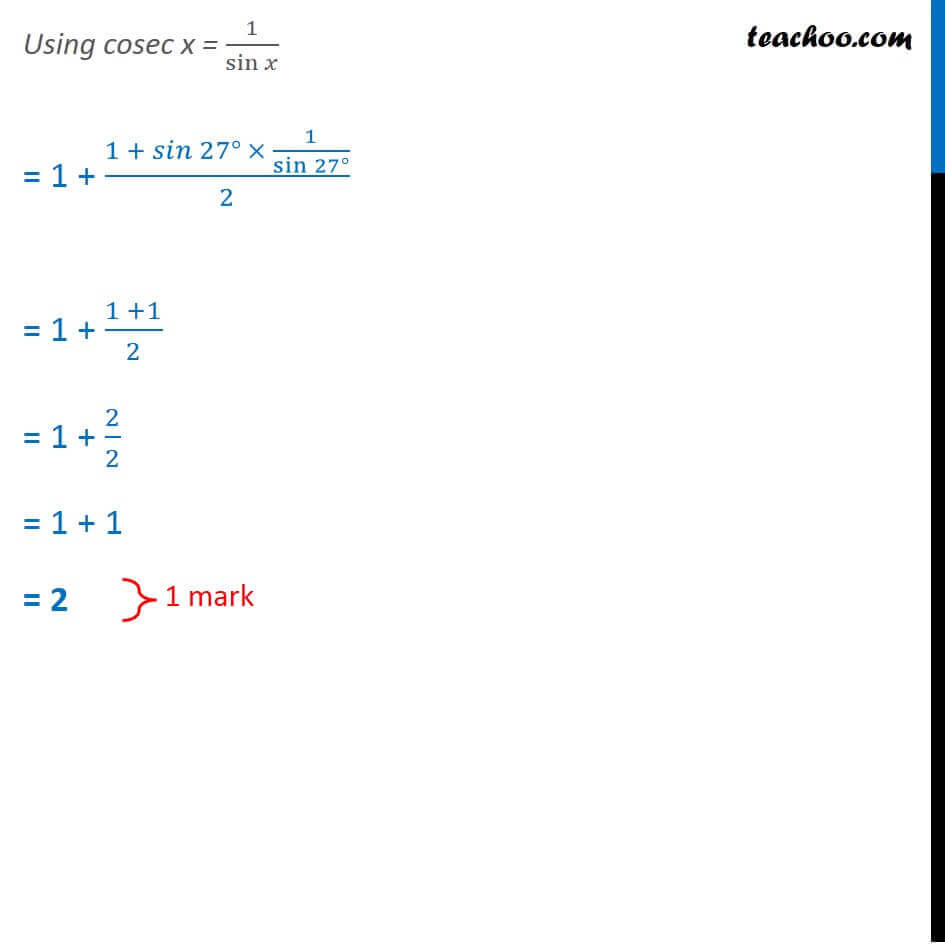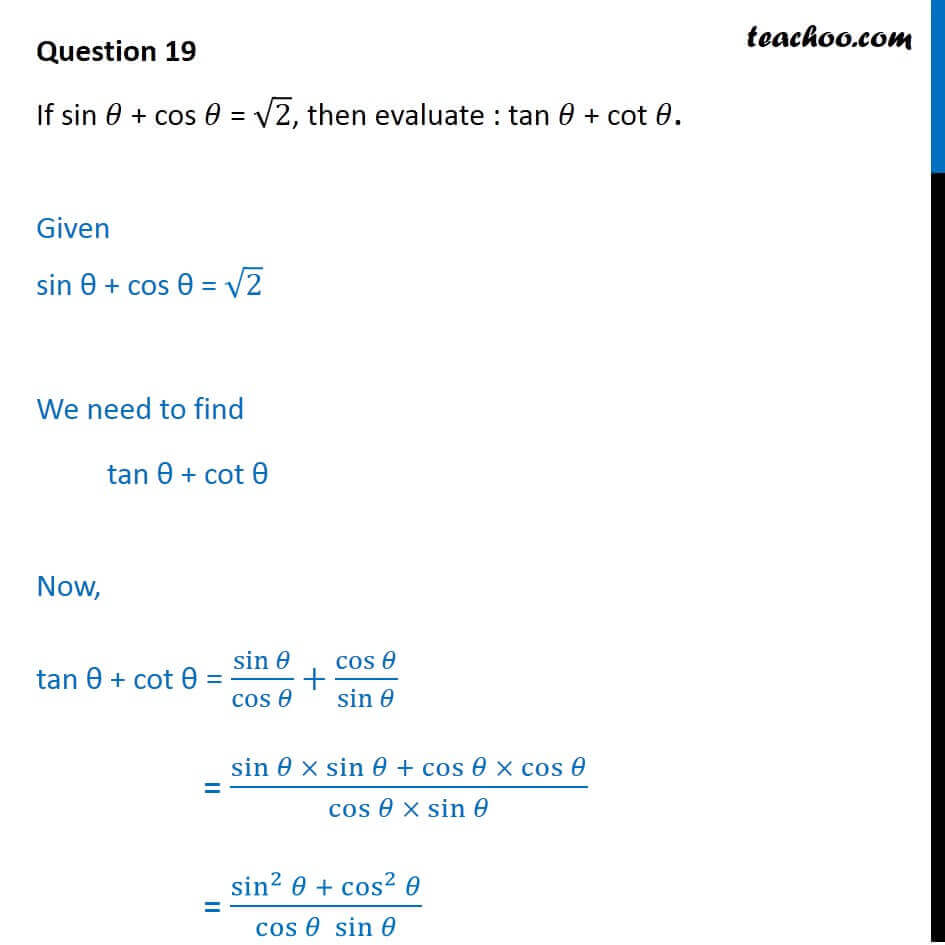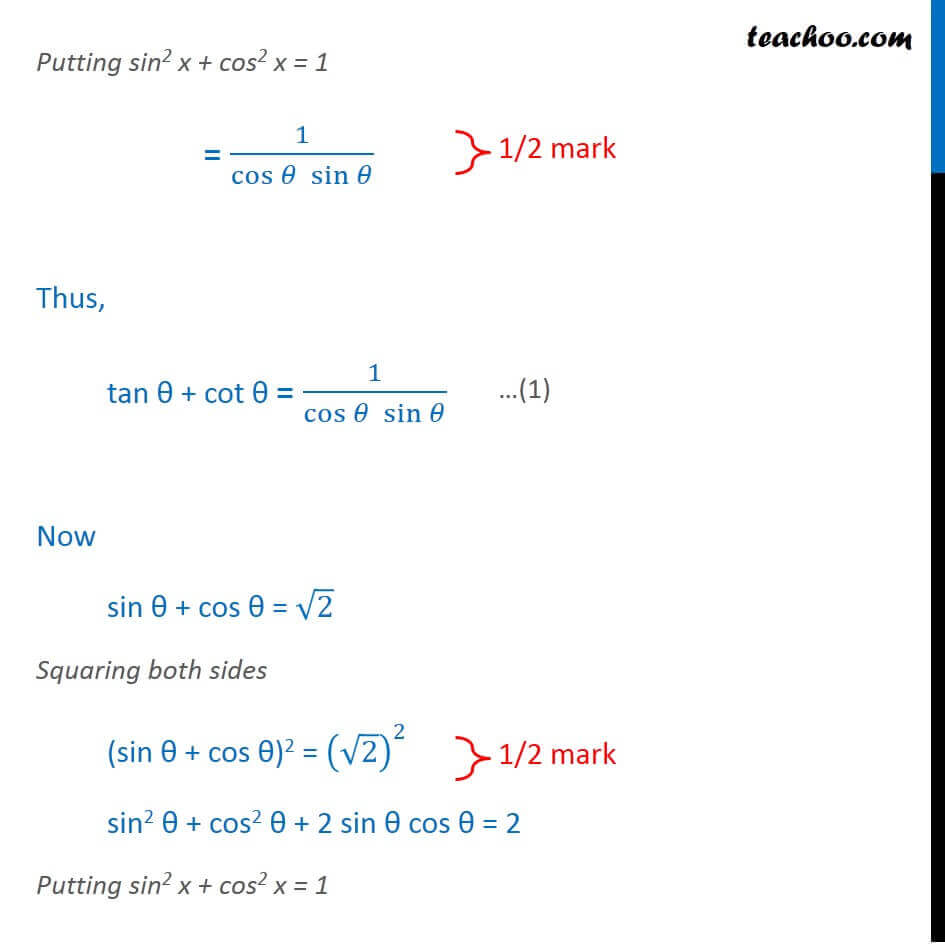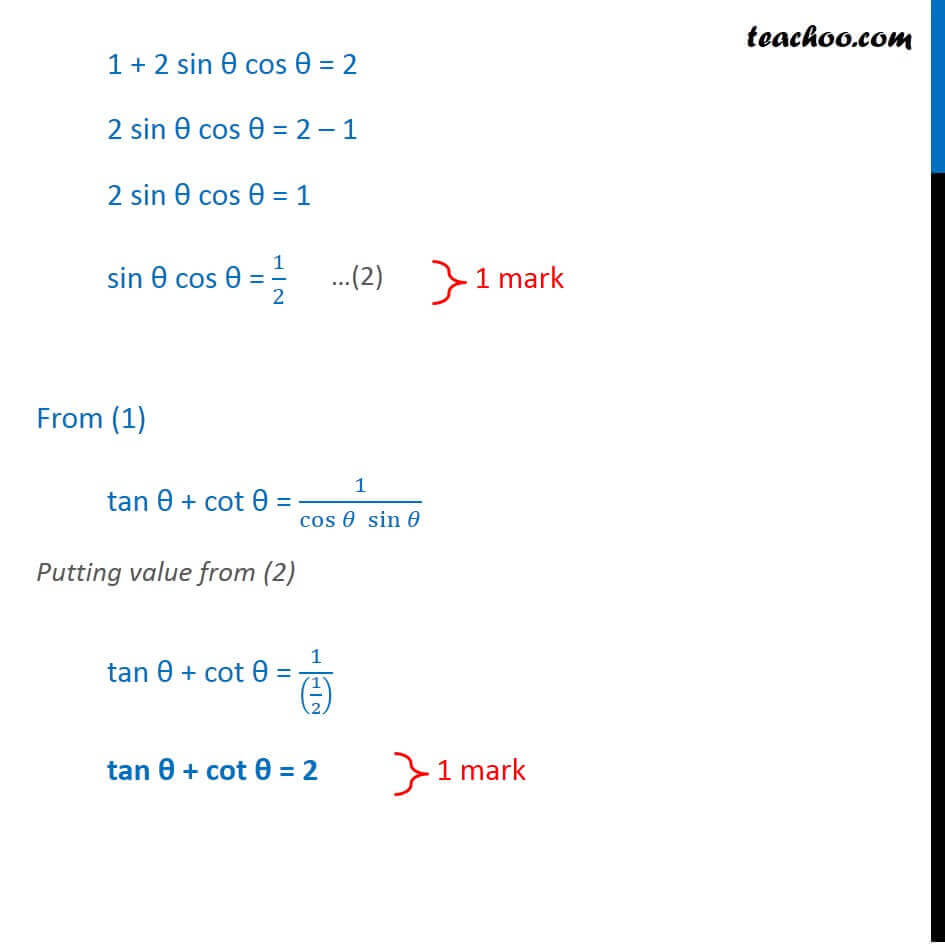Learn in your speed, with individual attention - Teachoo Maths 1-on-1 Class

### Transcript

Question 19 Evaluate: (cosec^2⁡〖63°〗 + tan^2⁡〖24°〗)/(cot^2⁡〖66°〗 + sec^2⁡〖27°〗 ) + (sin^2⁡〖63°〗 + cos⁡〖63°〗 sin⁡〖27°〗 + sin⁡〖27°〗 sec⁡〖63°〗)/(2(cosec^2⁡〖65°〗 − tan^2⁡〖25°〗)) (cosec^2⁡〖63°〗 + tan^2⁡〖24°〗)/(cot^2⁡〖66°〗 + sec^2⁡〖27°〗 ) + (sin^2⁡〖63°〗 + cos⁡〖63°〗 sin⁡〖27°〗 + sin⁡〖27°〗 sec⁡〖63°〗)/(2(cosec^2⁡〖65°〗 − tan^2⁡〖25°〗)) Theory We know that we have to use sin (90 – θ) = cos θ sin becomes cos cos becomes sin tan becomes cot cot becomes tan cosec becomes sec sec becomes cosec So, we need to form pairs Theory This will become sin2 63° + cos2 63° And we can use sin2 x + cos2 x = 1 This will become sin 27° × cosec 27° And we put cosec x = 1/sin⁡𝑥 (〖𝒄𝒐𝒔𝒆𝒄〗^𝟐⁡〖𝟔𝟑°〗 + 〖𝒕𝒂𝒏〗^𝟐⁡〖𝟐𝟒°〗)/(〖𝒄𝒐𝒕〗^𝟐⁡〖𝟔𝟔°〗 + 〖𝒔𝒆𝒄〗^𝟐⁡〖𝟐𝟕°〗 ) + (〖𝒔𝒊𝒏〗^𝟐⁡〖𝟔𝟑°〗 + 𝒄𝒐𝒔⁡〖𝟔𝟑°〗 𝐬𝐢𝐧⁡〖𝟐𝟕°〗 + 𝒔𝒊𝒏⁡〖𝟐𝟕°〗 𝒔𝒆𝒄⁡〖𝟔𝟑°〗)/(𝟐(〖𝒄𝒐𝒔𝒆𝒄〗^𝟐⁡〖𝟔𝟓°〗 − 〖𝒕𝒂𝒏〗^𝟐⁡〖𝟐𝟓°〗)) Denominator will become tan^2⁡〖24°〗+cosec^2⁡〖63°〗 and get cancelled by numerator Denominator will become cosec^2⁡〖65°〗 − cot^2⁡〖65°〗 And we can use cosec2 x – cot2 x = 1 (cosec^2⁡〖63°〗 + tan^2⁡〖24°〗)/(cot^2⁡〖66°〗 + sec^2⁡〖27°〗 ) + (sin^2⁡〖63°〗 + cos⁡〖63°〗 sin⁡〖27°〗 + sin⁡〖27°〗 sec⁡〖63°〗)/(2(cosec^2⁡〖65°〗 − tan^2⁡〖25°〗)) Using cos θ = sin (90 – θ) sin θ = cos (90 – θ) tan θ = cot (90 – θ) cot θ = tan (90 – θ) sec θ = cosec (90 – θ) cosec θ = sec (90 – θ) = (cosec^2⁡〖63°〗 + tan^2⁡〖24°〗)/(tan^2⁡〖(90° − 66°)〗 + cosec^2⁡〖(90° − 27°〗)) + (sin^2⁡〖63°〗 + cos⁡〖63°〗 〖cos 〗⁡〖(90° −27°)〗+ sin⁡〖27°〗 cosec⁡〖 (90° − 63°)〗)/2(cosec^2⁡〖65°〗 −〖 cot〗^2⁡〖(90° − 25°〗)) = (cosec^2⁡〖63°〗 + tan^2⁡〖24°〗)/(tan^2⁡〖(24°)〗 + cosec^2⁡〖(63°〗)) + (sin^2⁡〖63°〗 + cos⁡〖63°〗 cos⁡〖 (63°)〗+ sin⁡〖27°〗 cosec⁡〖(27°)〗)/2(cosec^2⁡〖65°〗 −〖 cot〗^2⁡〖(65°〗)) = 1 + (sin^2⁡〖63°〗 + cos^2⁡〖63°〗+ sin⁡〖27°〗 cosec⁡〖27°〗)/2(cosec^2⁡〖65°〗 −〖 cot〗^2⁡〖65°〗 ) Using cosec2 x – cot2 x = 1 = 1 + (〖𝒔𝒊𝒏〗^𝟐⁡〖𝟔𝟑°〗 + 〖𝒄𝒐𝒔〗^𝟐⁡〖𝟔𝟑°〗+ sin⁡〖27°〗 cosec⁡〖27°〗)/2(1) Using sin2 x + cos2 x = 1 = 1 + (1 + 𝑠𝑖𝑛⁡〖27°〗 𝑐𝑜𝑠𝑒𝑐⁡〖27°〗)/2 Using cosec x = 1/sin⁡𝑥 = 1 + (1 + 𝑠𝑖𝑛⁡〖27°〗 × 1/sin⁡〖27°〗 )/2 = 1 + (1 +1)/2 = 1 + 2/2 = 1 + 1 = 2 Question 19 If sin 𝜃 + cos 𝜃 = √2, then evaluate : tan 𝜃 + cot 𝜃. Given sin θ + cos θ = √2 We need to find tan θ + cot θ Now, tan θ + cot θ = sin⁡𝜃/cos⁡𝜃 +cos⁡𝜃/sin⁡𝜃 = (sin⁡𝜃 × sin⁡𝜃 + cos⁡𝜃 × cos⁡𝜃)/(cos⁡𝜃 × sin⁡𝜃 ) = (sin^2⁡𝜃 + cos^2⁡𝜃)/(cos⁡𝜃 sin⁡𝜃 ) Putting sin2 x + cos2 x = 1 = 1/(cos⁡𝜃 sin⁡𝜃 ) Thus, tan θ + cot θ = 1/(cos⁡𝜃 sin⁡𝜃 ) Now sin θ + cos θ = √2 Squaring both sides (sin θ + cos θ)2 = (√2)^2 sin2 θ + cos2 θ + 2 sin θ cos θ = 2 Putting sin2 x + cos2 x = 1 1 + 2 sin θ cos θ = 2 2 sin θ cos θ = 2 – 1 2 sin θ cos θ = 1 sin θ cos θ = 1/2 From (1) tan θ + cot θ = 1/(cos⁡𝜃 sin⁡𝜃 ) Putting value from (2) tan θ + cot θ = 1/((1/2) ) tan θ + cot θ = 2